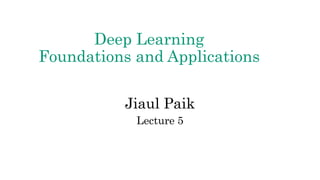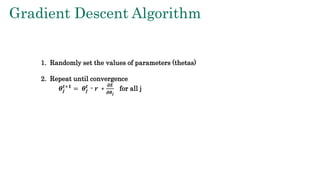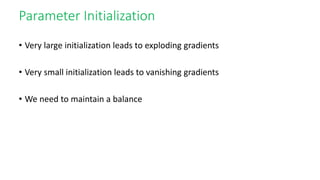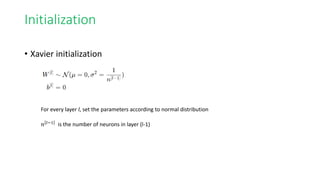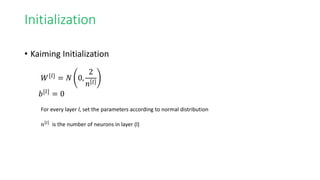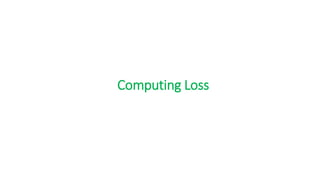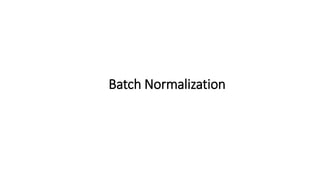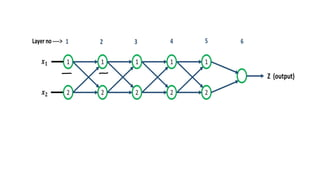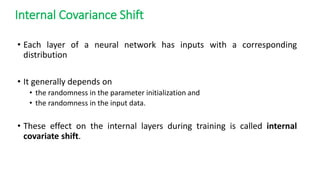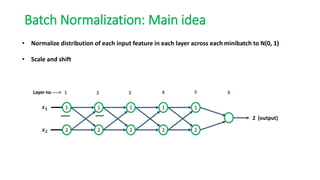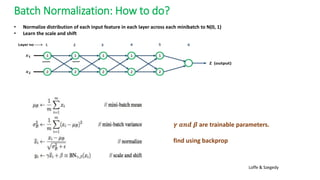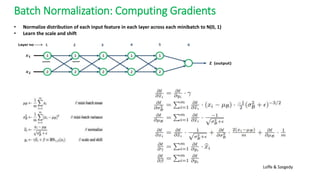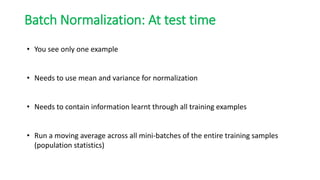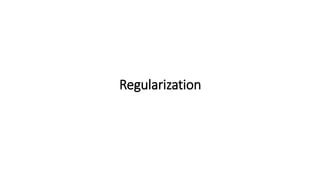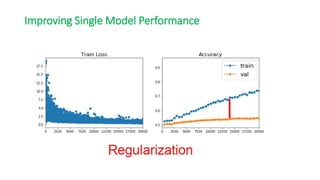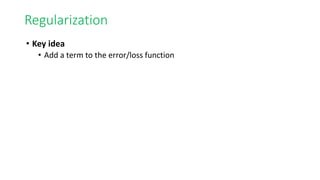1 de 20

### lecture-05.pptx

1. Deep Learning Foundations and Applications Jiaul Paik Lecture 5
2. Gradient Descent Algorithm 1. Randomly set the values of parameters (thetas) 2. Repeat until convergence 𝜽𝒋 𝒕+𝟏 = 𝜽𝒋 𝒕 - 𝒓 ∗ 𝝏𝑬 𝝏𝜽𝒋 for all j
3. Parameter Initialization • Very large initialization leads to exploding gradients • Very small initialization leads to vanishing gradients • We need to maintain a balance
4. Initialization • Xavier initialization For every layer l, set the parameters according to normal distribution 𝑛 𝑙−1 is the number of neurons in layer (l-1)
5. Initialization • Kaiming Initialization For every layer l, set the parameters according to normal distribution 𝑛 𝑙 is the number of neurons in layer (l) 𝑊[𝑙] = 𝑁 0, 2 𝑛 𝑙 𝑏[𝑙] = 0
6. Computing Loss
7. Cross Entropy
8. Batch Normalization
9. Internal Covariance Shift • Each layer of a neural network has inputs with a corresponding distribution • It generally depends on • the randomness in the parameter initialization and • the randomness in the input data. • These effect on the internal layers during training is called internal covariate shift.
10. Batch Normalization: Main idea • Normalize distribution of each input feature in each layer across each minibatch to N(0, 1) • Scale and shift
11. Batch Normalization: How to do? • Normalize distribution of each input feature in each layer across each minibatch to N(0, 1) • Learn the scale and shift 𝜸 𝒂𝒏𝒅 𝜷 are trainable parameters. find using backprop Loffe & Szegedy
12. Batch Normalization: Computing Gradients • Normalize distribution of each input feature in each layer across each minibatch to N(0, 1) • Learn the scale and shift Loffe & Szegedy
13. Batch Normalization: At test time • You see only one example • Needs to use mean and variance for normalization • Needs to contain information learnt through all training examples • Run a moving average across all mini-batches of the entire training samples (population statistics)
14. Regularization
15. Improving Single Model Performance
16. Regularization • Key idea • Add a term to the error/loss function
17. Regularization • Key idea • Add a term to the error/loss function
18. Regularization • Key idea • Add a term to the error/loss function
19. Regularization • Key idea • Add a term to the error/loss function